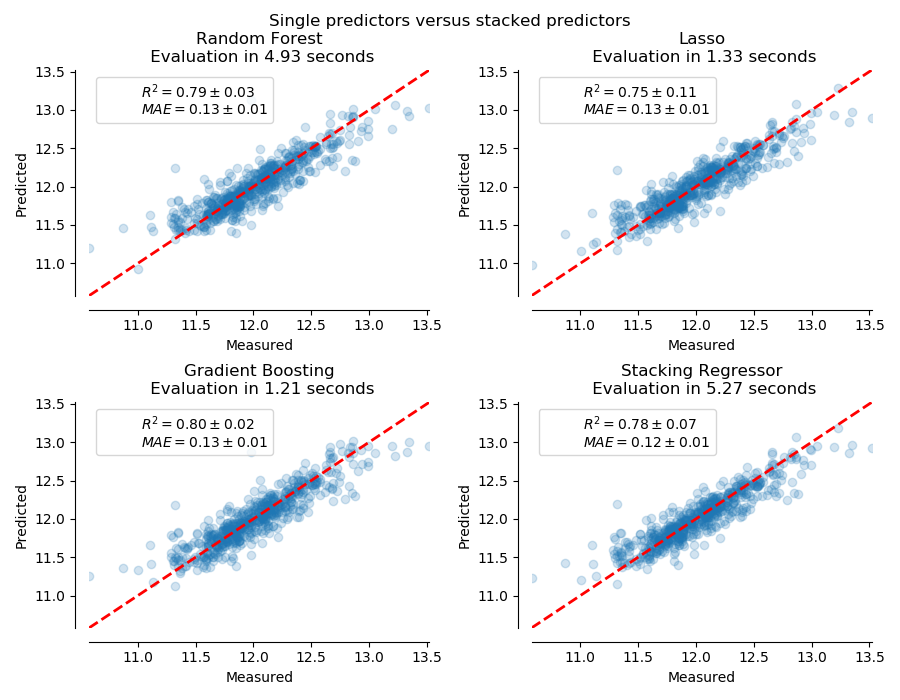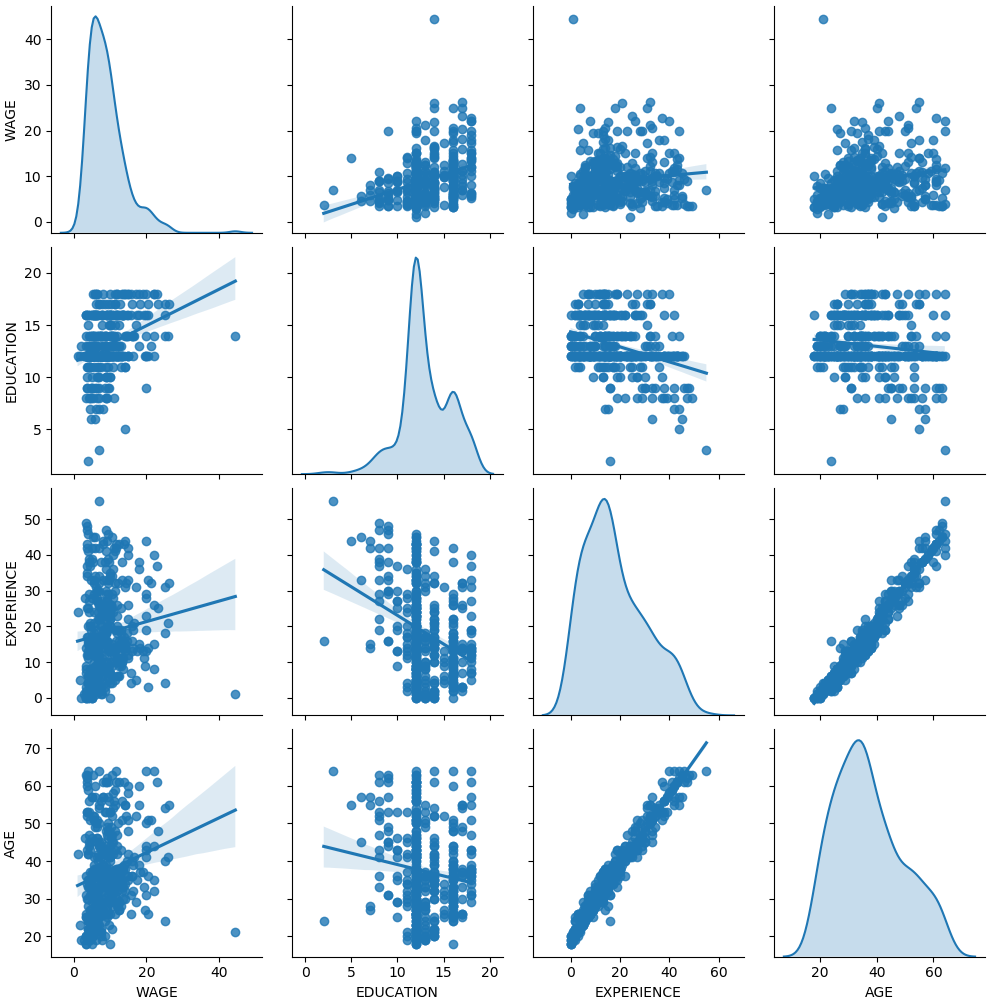# sklearn.compose.make_column_transformer¶

sklearn.compose.make_column_transformer(*transformers, **kwargs)

[源码]

*transformers tuples

transformer {‘drop’, ‘passthrough’} or estimator

columns str, array-like of str, int, array-like of int, slice, array-like of bool or callable

remainder {‘drop’, ‘passthrough’} or estimator, default=’drop’

sparse_threshold float, default=0.3

n_jobs int, default=None

verbose bool, default=False

ct ColumnTransformer

>>> from sklearn.preprocessing import StandardScaler, OneHotEncoder>>> from sklearn.compose import make_column_transformer>>> make_column_transformer(...     (StandardScaler(), ['numerical_column']),...     (OneHotEncoder(), ['categorical_column']))ColumnTransformer(transformers=[('standardscaler', StandardScaler(...),                                 ['numerical_column']),                                ('onehotencoder', OneHotEncoder(...),                                 ['categorical_column'])])

## sklearn.compose.make_column_transformer使用示例¶